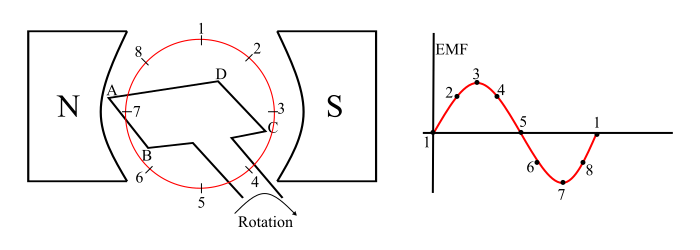# Electric Generator: Construction and Working Principle

The electric generator is an electromechanical energy conversion device, which converts mechanical energy into electrical energy.

## Construction of Electric Generator

An electric generator has following main parts −

• Magnetic Field System

• Armature Core

• Armature Winding

• Yoke### Magnetic Field System

The magnetic field system is used to produce uniform magnetic field in the electric generator within which the armature rotates. The field system may be composed of either permanent magnets (small generators) or electromagnets (in large generators). Generally, the field system of a practical electric generator consists of poles on which the field coils are mounted. When field coils are energised, a magnetic field produces within the generator.

### Armature Core

The armature core is mounted on the machine shaft and rotates in the magnetic field of field poles. It consists of slotted soft iron laminations that are stacked to form a cylindrical core. The laminations are insulated from each other by a thin film of varnish. The core is made laminated to reduce the eddy current loss. The armature conductors are placed into the slots, made on the outer periphery of the armature core.

### Armature Winding

The slots of the armature core hold insulated conductors, which are connected in a suitable manner. This is called as armature winding. This is the winding in which the working EMF induces.

### Yoke

The circular frame of the electric generator is called yoke. The yoke of electric generator is usually made of solid cast steel. The yoke increases the mechanical strength of the generator. It also provides path to the magnetic flux produced by the field winding.

## Working of Electric Generator

Consider a simple loop electric generator (as shown in the figure), in this a single turn loop ‘ABCD’ is rotating in clockwise in a uniform magnetic field with a constant speed. When the loop rotates, the magnetic flux linking the coil sides ‘AB’ and ‘CD’ changes continuously. This change in flux linkage induces an EMF in coil sides and the induced EMF in one coil side adds the induced EMF in the other.The EMF induced in an electric generator can be explained as follows −

• When the loop is in position-1, the generated EMF is zero because, the movement of coil sides is parallel to the magnetic flux.

• When the loop is in position-2, the coil sides are moving at an angle to the magnetic flux and hence, a small EMF is generated.

• When the loop is in position-3, the coil sides are moving at right angle to the magnetic flux, therefore the generated EMF is maximum.

• When the loop is in position-4, the coil sides are cutting the magnetic flux at an angle, thus a reduced EMF is generated in the coil sides.

• When the loop is in position-5, no flux linkage with the coil side and are moving parallel to the magnetic flux. Therefore, no EMF is generated in the coil.

• At the position-6, the coil sides move under a pole of opposite polarity and hence the polarity of generated EMF is reversed. The maximum EMF will generate in this direction at position-7 and zero when at position-1. This cycle repeats with revolution of the coil.

In this way, the EMF is generated in an electric generator, where the mechanical energy of rotation is converted into electrical energy.

## Losses in Electric Generator

The losses in an electric generator can be classified into three categories as −

### Copper Losses

These losses occur due to ohmic resistance of the windings. The copper losses are the variable losses because these are the function of current in the windings.

Copper Losses,

$$P_{cu}=I^{2}R\:Watts$$

### Iron or Core Losses

The iron or core losses occur in armature core of the electric generator because the armature rotates in magnetic field. They are of two types −

Hysteresis Loss (Ph)

The hysteresis loss occurs in the armature core because the armature is subjected to magnetic reversal. It is given by the equation,

$$p_{h}=K_{h}B_{max}^{1.6}f^{2}t^{2}V\:Watts$$

Eddy Current Loss (Pe)

When the armature rotates in the magnetic field, an EMF is induced in the core of the armature which circulates eddy currents in the armature core. The power loss due to these eddy current is known as eddy current loss.

$$p_{e}=K_{e}B_{max}^{2}f^{2}t^{2}V\:Watts$$

### Mechanical Losses

The mechanical losses are due to friction and windage.

• Friction Loss – For Example bearing friction, brush friction etc.

• Windage Loss – It is due to air friction of rotating armature.